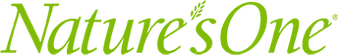How to Calculate Birdhouse Roof Angles | Family life

# How to Calculate Birdhouse Roof Angles

-

#### Things You\’ll Need

• Pencil

• Paper

• Ruler

• Scientific calculatorA birdhouse roof\’s angles need to be exact. Image Credit: Jupiterimages/Creatas/Getty Images

A completed birdhouse may look like a seamless single structure, but is really made of a set of precisely fitted pieces to eliminate any gaps in the roof and walls. Carpenters use a miter saw to produce cuts at exact angles and fit these pieces together. Calculating the angles of the birdhouse's roof will prevent you from wasting wood from incorrect cuts. Angle calculation involves basic geometry and trigonometry formulas.

Video of the Day

## Step 1

Draft the diagram of your birdhouse roof viewed from the side as a triangle and write the desired length of the base. For an example, assume your birdhouse roof's base is 20 inches long.

## Step 2

Draw and label a line bisecting the triangle. Mark its length from the tip of the triangle to its intersection with the base; this is the height of the roof. Assume the height of the roof to be five inches in this example. Label the two new bases as 10 inches each, half the length of the original 20 inches.

## Step 3

Take the square of the length of a base, 100, and add it to the square of the height, 25. Adding these numbers gives you 125. Take the square root of 125 to determine the length of the hypotenuse, or the roof. You will get 11.18 inches as a result.

## Step 4

Divide the height, 5, by the length of the base, 10, to get 0.5. Enter this value into your calculator and press the key labeled "tan-1" or "arctan." You should get 26.57 as a result. This is the angle of the roof from the horizontal. The tangent function calculates side lengths from angles, whereas the inverse tangent function calculates angles from side lengths.

## Step 5

Add 90 and 26.57 to get 116.57. Subtract this from 180 to get 63.43 as the roof's angle from the vertical. Label all angles.

#### Tip

Trigonometric functions will work as long as you have the roof divided into right triangles. Round decimals to the hundredths place, or two digits past the decimal point.

### Tips for a Budget-Friendly Road Trip